# 2018-2019 BPHO英国物理奥赛第一轮第二部分真题及解析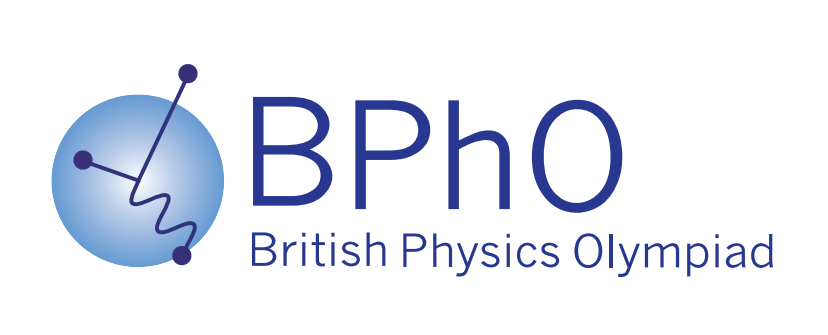BPhO Round 1

Section 2

16th November 2018

This question paper must not be taken out of the exam room

Instructions

Time: 5 minutes reading time (NO writing) and 1 hour 20 minutes for writing on this section.

Questions: Only two questions out of the fifive questions in Section 2 should be attempted.

Each question contains independent parts so that later parts should be attempted even if  earlier parts are incomplete.

Working: Working, calculations, explanations and diagrams, properly laid out, must be shown for full credit. The fifinal answer alone is not suffificient. Writing must be clear. If derivations are required, they must be mathematically supported, with any approximations stated and justifified.

Marks: Students are recommended to spend about 40 minutes on each question. Each question in Section 2 is out of 25, with a maximum of 50 marks from two questions only.

Instructions: You are allowed any standard exam board data/formula sheet.

Calculators: Any standard calculator may be used, but calculators cannot be programmable and must not have symbolic algebra capability.

Solutions: Answers and calculations are to be written on loose paper or in examination booklets. Graph paper and formula sheets should also be made available. Students should ensure that their name  and their school/college are clearly written on each and every answer sheet.

Setting the paper: There are two options for sitting BPhO Round 1:

a.Section 1 and Section 2 may be sat in one session of 2 hours 40 minutes plus 5 minutes reading time(for Section 2). Section 1 should be collected in after 1 hour 20 minutes and then Section 2 given out.

b.Section 1 and Section 2 may be sat in two sessions on separate occasions, with 1 hour 20 minutes

plus 5 minutes reading time allocated for Section 2. If the paper is taken in two sessions on separate occasions, Section 1 must be collected in after the fifirst session and Section 2 handed out at the beginning of the second session.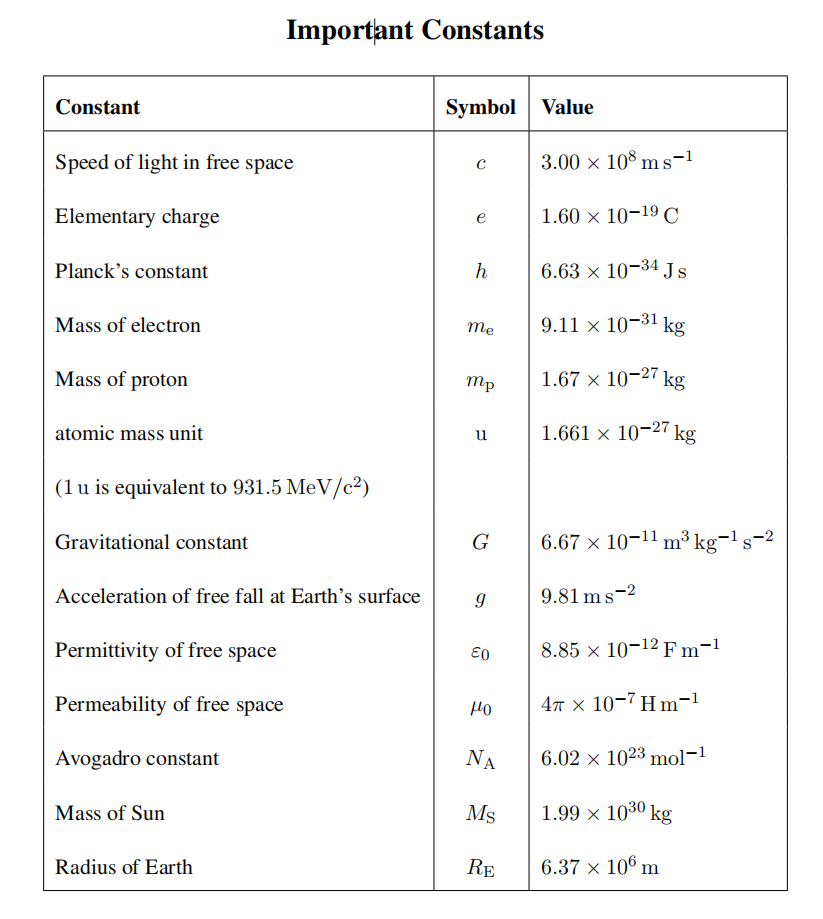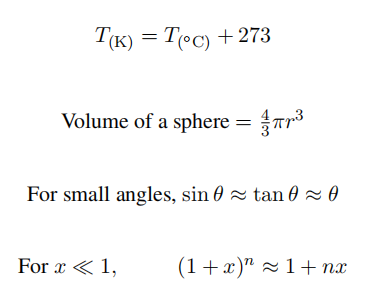Section 2

Question 2

a) A planet has a radius R and the gravitational fifield strength at its surface is g. A satellite is placed in a circular orbit of radius r around the planet.

Obtain an expression for T, the period of orbit of the satellite, in terms of R, g and r, explaining the relevant physics in each of your steps.

b) Both the Earth and Jupiter can be assumed to have circular orbits about the Sun. Jupiter has an orbital period, TJupiter = 11.9 years. What is the orbital radius of Jupiter in au?

Note: an au (astronomical unit) is the average distance between the Earth and the Sun.

(c) The density of the Earth changes sharply between the core and the mantle. By taking just two suitable values of the density of the core and mantle of the Earth from the graph in Figure 1, estimate a value of the mass of the Earth using this data. Your values and calculation must be shown.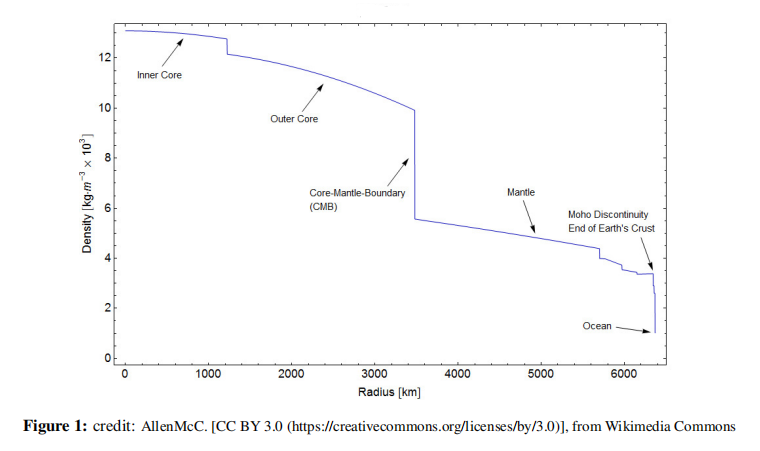d) A planet of mass Mp has an orbiting moon of mass Mm. The mass of the moon is about 1/8 the mass of the planet.

(i) Sketch a set of equipotential lines for the planet-moon system. Ignore the orbital motion of the moon. Without detailed calculation, indicate on your sketch the relative positions of the neutral point (a point of zero fifield strength) and the centre of mass of the planet-moon system.

A rocket of mass m is launched from the planet. The rocket engine is used very brieflfly at take-off when it is close to the planet’s surface, and it almost immediately reaches speed v, after which the fuel is exhausted and there is no further propulsion. It is then in free-fall and will have just enough energy to reach the moon. Assume that you can ignore relative motion of the planet and moon, and ignore air resistance.

Obtain expressions and calculate values for:

(ii) The distance, d, from the planet’s centre, where the speed of the rocket is lowest.

(iii) The launch speed v of the rocket from the planet, in terms of the variables Mp, Mm, the radius of the planet, Rp, and the separation of the centres of the moon and planet, D.

For this question part, you may take Mp = 6.00 × 1024 kg, Mm = 7.50 × 1023 kg,

D = 3.84 × 108 m.

e) The Earth can be considered to consist of concentric spheres of material, the outer sphere being the crust with a density of 2500 kg m -3 . If the mass of the Earth is ME = 6.0 × 1024 kg, what will be the fractional change in the gravitational fifield strength when descending to the bottom of a gold mine, a distance d = 3000 m below the surface of the Earth?

Hint: the fifield inside a hollow spherical shell is zero.

f) A planetary sized object similar to the Earth was formed from a very large spherical cloud of interstellar dust collapsing under gravity to form a spherical body of uniform density ρ and radius R

(i) Find an expression for the change in gravitational potential energy as the radius of the proto

planet increases from r to r + δr by the formation of a thin spherical shell of material of mass δm.

(ii) Now derive an expression for the total transfer of gravitational potential energy in the formation of the planet. Use the following terms: the gravitational constant G, and the fifinal radius and mass, R and M respectively.

(iii) If all of this energy was used to heat the planet formed at a very low initial temperature, estimate the temperature that the planet would have reached.

For this planet, take R = 6400 km, M = 4.0 × 1026 kg, and the average specifific heat capacity of the material of the planet is 700 J kg -1-1.

Question 3

a) A particle is moving in a horizontal circle and is kept in its path by a string tied to a point at a height h above the centre of the circle.

(i) Find the period of rotation in terms of h and the gravitational fifield strength g, explaining the physics of your derivation in words.

(ii) If the tension in the string is three times the weight of the particle, fifind the length of the string ` , in terms of h.

b) (i) A particle of mass m is suspended by a thin elastic string of natural length a in which a tension mg would produce an extension a. Write down an expression for the period of oscillation of the mass in terms of a and g.

(ii) Two particles of equal mass m on a smooth horizontal table are connected by the same thin elastic string of natural length a. The particles are held at rest at a distance 3a apart.

i. Describe their motions between the time they are released and when they collide.

ii. If the particles are released simultaneously, calculate the time elapsed before they collide, given a = 0.20 m.

c) A piece of elastic cord of length ` and cross-sectional area A is stretched to twice its natural length.

Assuming that the total volume remains constant, and that cross-sectional area is uniform along its length, and the Young’s modulus E for the material remains constant,

(i) obtain an expression for the fifinal tension in terms of E and A.

(ii) Show that the work done in stretching the elastic is given by E`A(1 ln 2)

d) The behaviour of the boxing training ball shown in Figure 2 can be modelled as two identical vertical springs attached to a mass, m. The springs each have a natural length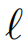, and a spring constant k, such that an applied force of mg doubles the length of spring to 2`. The top

and bottom springs are attached to the ceiling and flfloor respectively in a room of height 4`.

Assume the radius of the ball, r.

(i) Determine the equilibrium position of the ball below the ceiling in terms of.

(ii) The ball is given a small vertical displacement and released. Find an expression for the period Tv for the vertical oscillations.

(iii) The ball is given a small horizontal displacement.

Using a suitable approximation provide an expression for the horizontal force Fh exerted on

the ball by the springs.

(iv) Find an expression for the period of the horizontal oscillations Th and evaluate the ratio Th/Tv(Image credit: Kangrui Sports http://kangruisport.com/sport/punchingbags )

Question 4

a) (i) A tall cylinder of circular cross sectional area A and height h is fifilled with a liquid of uniform density ρ.

i. Derive an expression for the pressure P at the bottom of the liquid in terms of the variables ρ, g and h. Ignore atmospheric pressure.

ii. Show that the units for each side of your derived equation are the same.

iii. What would your result be if the liquid changed in density linearly from ρt at the top to ρb at the bottom of the cylinder?

iv. If you used your formula for the pressure at ground level, at the bottom of the Earth’s atmosphere, which has a height of about 200 km, it would give an answer which is very far from correct. Explain why.

(ii) A water tank which sits on the ground is kept full of water to a depth of h. Water is issuing horizontally from a hole which is in the sidewall of the tank, a distance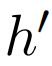below the surface.

Determine the maximum horizontal distance H from the hole in the can where the jet of water strikes the flfloor, by using arguments which assume only

i. conservation of mechanical energy, assuming the water falls down through

height,

ii. conservation of momentum (using the pressure at depth).

(b) An empty, hollow cuboid container of mass M and height h, width a, depth b, is suspended upright from a thin cable in a liquid of density ρ` , as shown in Figures 3a and 3b.

(i) Find an expression for the tension, T, in the cable by considering the force due to the pressure of the liquid on the top of the container, and the force due to the pressure of the liquid on the bottom of the container.

(ii) The cuboid container now has a small hole pierced in the bottom surface and the liquid leaks in until the air now confifined to the top part of the container, occupying a fraction f of the volume, with a fraction (1 f) of the volume inside now being fifilled with liquid. The pressure of the atmosphere at the surface of the liquid is P0 and the density of the liquid is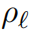. Obtain an expression for the depth, h0 of the bottom surface of the container in terms of P0,, g and h.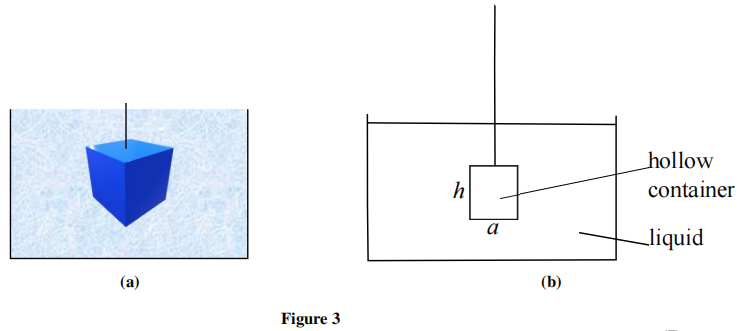c) A rod of length λ and material density ρr is pivoted by a hinge at a point on the wall of a container which has liquid of density, below the level of the pivot point. The rod flfloats without touching the bottom of the container. If a fraction λ/n of the rod is above the surface, determine n in terms of ρr and.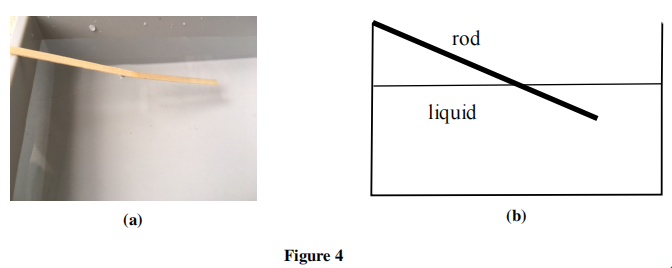d) A solid circular cone of height h, mass m, radius of base, r, is flfloating in a liquid of densitywith its axis vertical and vertex downwards, as shown in Figure 5. In the liquid, a height h/n is submerged below the surface of the liquid.

(i) Obtain an expression relating the mass of the cone to the fraction submerged,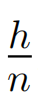, in terms of r, h, n andThe volume of a circular cone is given by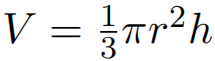(ii) A weight equal to the weight of the cone is placed on the base whilst it is flfloating, and it sinks until the base is just level with the liquid, and then it rises again. For this condition,

show that

n 3+ n 2 + n = 7

You may neglect any effects of the inertia and viscosity of the liquid. Energy considerations may be helpful.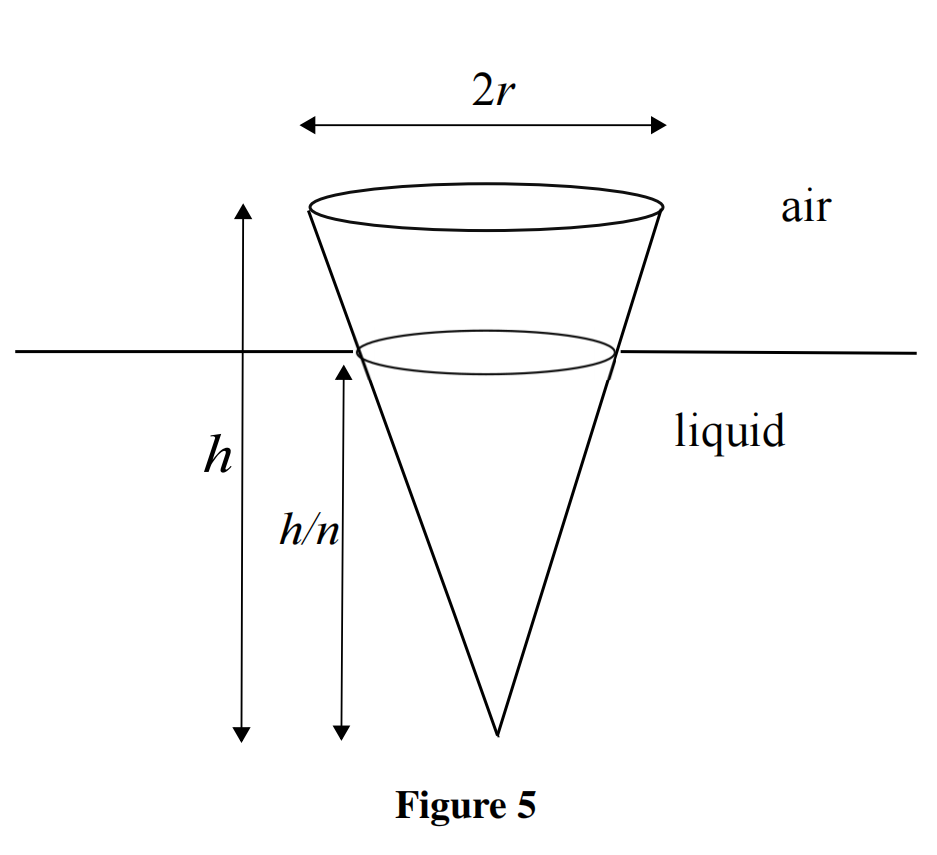Question 5

a) In the two circuits shown in Figures 6a and 6b below, a cell of e.m.f. E and negligible internal resistance is connected across a pair of resistors, R1 and R2. Resistor R1 is a length of uniform resistance wire, and R2 is a fifixed resistor.

(i) For the circuit shown in Figure 6a, sketch two graphs on the same axes showing how the potential V across R2 varies with position of the sliding contact on R1 for the cases

i. when R2  》 R1

ii. when R2 《  R1

(ii) Repeat part (i) above for the circuit shown in Figure 6b.

(iii) What are the relative advantages of each of these circuits when used to control the potential

difference across R2?b) A resistance network is shown in Figure 7.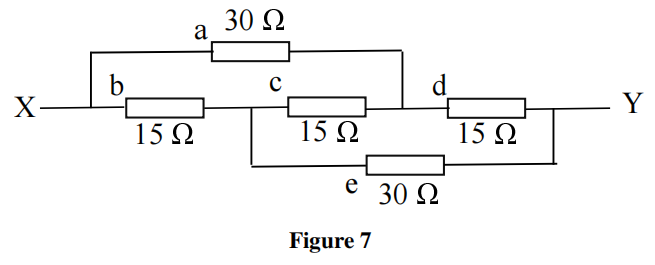(i) A total current of 10 mA flflows through the circuit. Determine the current in each of the

resistors, a to e.

(ii) Hence fifind the total resistance between X and Y

c) A strain gauge consists of a straight metal wire of length 0.30 m, diameter 0.028 mm and resistivity 5.0 × 107 Ω m. Calculate the resistance of the gauge when

(i) unstrained and

(ii) stretched by 1.0 mm. You may assume that the diameter of the wire does not change.

(iii) Calculate the resistance if the stretched wire maintained the same volume i.e. the diameter decreased in the process of stretching?

(iv) Four identical strain gauges as in c) (ii) above, labelled R1 to R4, are arranged in a bridge circuit as shown in Figure 8, with a cell of e.m.f 9.0 V and of negligible internal resistance. R1 and R4 are stretched by 1.0 mm. Assuming the internal resistance of the power supply is negligible, calculate the reading on the voltmeter (which can be considered to have an infifinite resistance).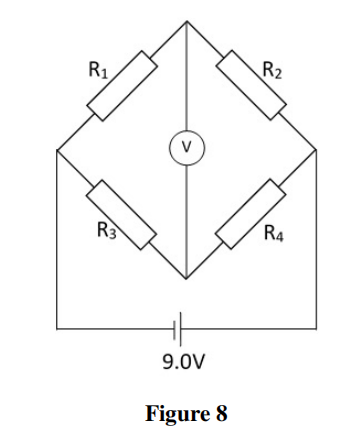d) The following network shown in Figure 9 is infifinite. Find an expression for the resistance between

A and B in as simple a form as possible, and determine the resistance when r1 = 4 Ω and r2 = 15 Ω. Hint: consider adding new resistors r1 and r2 at the left end of the circuit shown.e) The network shown in Figure 10 consists of an infifinite number of cells of e.m.f. ε1 and ε2 with corresponding internal resistances R1 and R2 respectively. A resistor R of value R = 1.0 Ω, is connected to the end of the series. If the values of the e.m.f.s and resistances are ε1 = 1.0 V, ε2 = 2.0 V, R1 = 4 Ω, R2 = 6 Ω, determine the value of the current flflowing through resistor R.Question 6

a) Two uncharged capacitors with values C1 and C2, are connected in series with a battery of e.m.f. V , and a switch. When the switch is closed there is a charge of Q1 and Q2 on the capacitors respectively.

(i) State the relationship between Q1 and Q2.

(ii) State expressions for the potential differences across each capacitor, V1 and V2

(iii) Hence derive an expression for the equivalent capacitance, Ceq produced by C1 and C2 in series.

(iv) Write down expressions for the total energy stored in the two capacitors, and the energy supplied by the battery, in terms of Ceq and V , and comment on the difference

b) A 15 nF parallel plate capacitor is connected across a 70 V battery, as in Figure 11. How much work must be done in order to double the plate separation

(i) with the battery disconnected,

(ii) with the battery connected?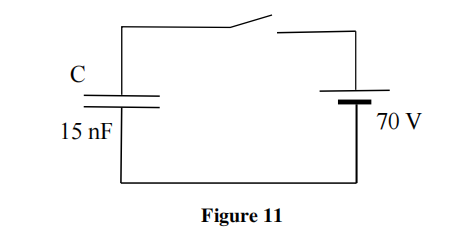c) (i) Two capacitors, of capacitance 2.0 µ F and 4.0 µ F, are each given a charge of 120 µ C. The positive sides are then connected, as are the negative sides. Calculate:

i. The new potential difference across the plates.

ii. The change in the energy stored.

(ii) A conducting metal sphere is insulated from the ground. It is initially charged by contact with a small metal disc which carries a charge q, so that the sphere gains charge Q. The metal disc is then again given a charge q, and is then touched on the sphere a second time. If this process is repeated indefifinitely, what would be the fifinal charge on the sphere, Qf ?

(iii) Three capacitors with leads AB, CD and EF of capacitances 1 µF, 2 µF and 3 µF respectively are each connected separately to a battery and charged to a potential of 12 V. The positively charged plates are A, C and E. The capacitors are joined in series so that B is connected to C, D is connected to E, and F is connected to A.

i.Calculate the charges on each of the capacitors, indicating the values and signs on a diagram.

ii. Sketch a graph to show the variation of potential around the circuit from A to F.

d) A pair of concentric conducting spheres have radii of 10 cm and 15 cm They are insulated from each other, and the potential difference between the spheres is 120 V. The spheres carry equal charges ±Q, with the inner sphere being positive..

(i) Sketch the shape of a graph of the electric fifield strength between r = 0 cm and r = 30 cm.

(ii) Sketch a graph of the potential between r = 0 cm and r = 30 cm.

(iii) Write down expressions for the potential on the inner charged sphere if the outer sphere were not present, and the potential for the outer charged sphere if the inner sphere were not present.

(iv) From these results, calculate the magnitude of the charge Q

e) An LED is to be lit with a constant current of 800 mA supplied by a charged capacitor, C, in series with a variable resistor, R, as shown in Figure 12. The diode has a forward bias of 2.7 V, which means that when the diode is conducting a current, it has this fifixed potential difference across it.

(i) What is the variation R(t) of the variable resistor against time, such that the constant current is maintained?

The initial potential difference across the capacitor is 5.0 V.

(ii) For how long would the LED remain lit if the value of the capacitor is 0.4 µ F?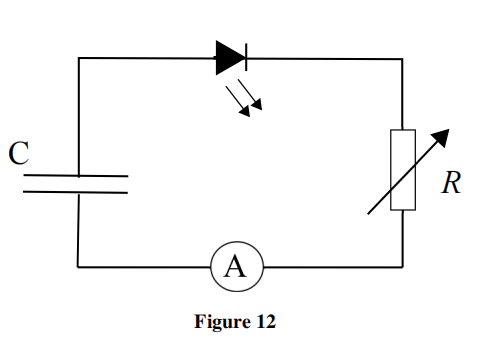END OF SECTION 2